Next: Exercises Up: Using partial derivatives for Previous: Purpose

## Background and Examples

The only new Maple procedure that needs to be introduced in this lab is the procedure for taking partial derivatives. Partial derivatives are needed to compute things such as gradients, tangent planes, and differentials.

You have seen in the past that there are two commands used to compute derivatives; diff and D. For example, to compute the derivative of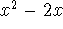, the following can be done:

```  > f1:= x^2+2*x;
```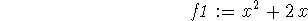```  > df1:=diff(f1,x);
```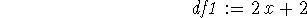```  > f2:= x -> x^2+2*x;
```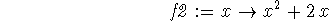```  > df2a:=diff(f2(x),x);
```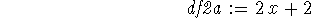```  > df2b:=D(f2);
```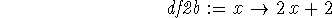Note that when using the D command, a function must be used. When using the diff command, a function or expression can be used. Therefore, you are always safe in defining functions, not expressions. Also note that out of the three derivatives, only the one computed using the D command remains a function, not an expression.

Now, to carry these commands over to deal with partial derivatives, everything remains the same except for one small change in the D command. When a function is defined in more than one variable, Maple takes note of the order in which the variables are listed. Therefore, if you wish to find the partial derivative with respect to the first variable, you would need to add the number one inside square brackets after the D, but before the function name. Here are some examples. Note again, that by using the D command, the resulting partial derivative is a function. This is useful when you need to substitute values into variables.

```  > g:= (x,y) -> ln(2*x+3*y);
```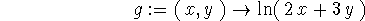```  > gx1:=diff(g(x,y),x);
``````  > gx2:=D(g);
```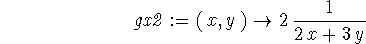```  > h:= (x,y,z) -> x^2+y^2-2*x*y*cos(z);
```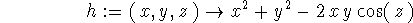```  > hz1:=diff(h(x,y,z),z);
``````  > hz2:=D(h);
```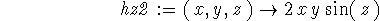Sean O Anderson
Tue Apr 15 11:51:21 EDT 1997